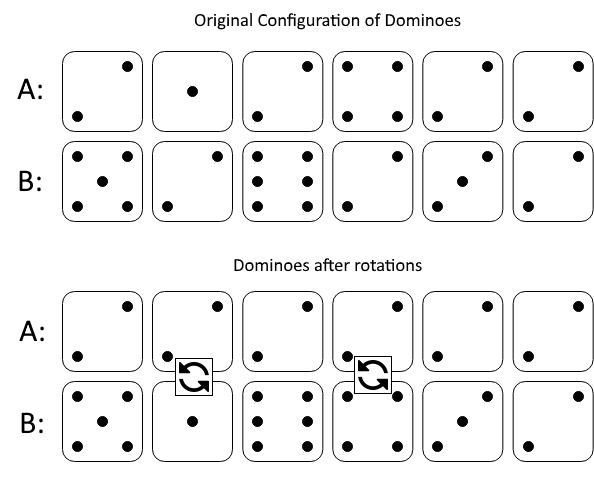# LeetCode: 1007. 行相等的最少多米诺旋转¶

## 1、题目描述¶输入：A = [2,1,2,4,2,2], B = [5,2,6,2,3,2]



输入：A = [3,5,1,2,3], B = [3,6,3,3,4]



• 1 <= A[i], B[i] <= 6
• 2 <= A.length == B.length <= 20000

## 2、解题思路¶

• 上下数字相同的数字超过1个，肯定无法变成相同的值
• 上下数字相同的数字为1个：
num, count = same.popitem()
if top[num] + bottom[num] - count == length:
return min(top[num], bottom[num]) - count
else:
return -1


• 上下数字没有相同的，遍历一遍所有的key，判断当前数字数量书否等于数组长度，等于则更新结果
from collections import defaultdict

class Solution:
def minDominoRotations(self, A: List[int], B: List[int]) -> int:
length = len(A)
top = defaultdict(int)
bottom = defaultdict(int)
same = defaultdict(int)
ans = length

for i in range(length):
top[A[i]] += 1
bottom[B[i]] += 1
if A[i] == B[i]:
same[A[i]] += 1

if len(same) >= 2:
return -1
elif len(same) == 1:
num, count = same.popitem()
if top[num] + bottom[num] - count == length:
return min(top[num], bottom[num]) - count
else:
return -1

for num, count in top.items():
if count + bottom[num] == length:
ans = min(count, bottom[num])

return ans if ans != length else -1